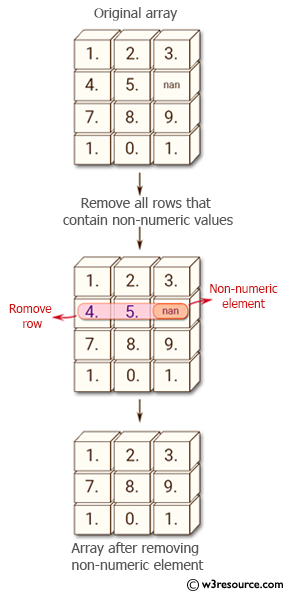﻿ NumPy: Remove all rows in a NumPy array that contain non-numeric values - w3resource# NumPy: Remove all rows in a NumPy array that contain non-numeric values

## NumPy: Array Object Exercise-91 with Solution

Write a NumPy program to remove all rows in a NumPy array that contain non-numeric values.

Pictorial Presentation:Sample Solution:

Python Code:

``````import numpy as np
x = np.array([[1,2,3], [4,5,np.nan], [7,8,9], [True, False, True]])
print("Original array:")
print(x)
print("Remove all non-numeric elements of the said array")
print(x[~np.isnan(x).any(axis=1)])
```
```

Sample Output:

```Original array:
[[  1.   2.   3.]
[  4.   5.  nan]
[  7.   8.   9.]
[  1.   0.   1.]]
Remove all non-numeric elements of the said array
[[ 1.  2.  3.]
[ 7.  8.  9.]
[ 1.  0.  1.]]
```

Python Code Editor:

Have another way to solve this solution? Contribute your code (and comments) through Disqus.

What is the difficulty level of this exercise?

Test your Python skills with w3resource's quiz

﻿

## Python: Tips of the Day

Set comprehension:

```>>> m = {x ** 2 for x in range(5)}
>>> m
{0, 1, 4, 9, 16}
```